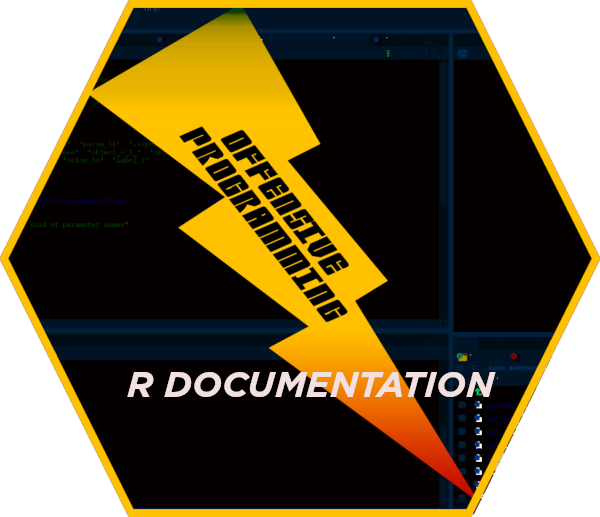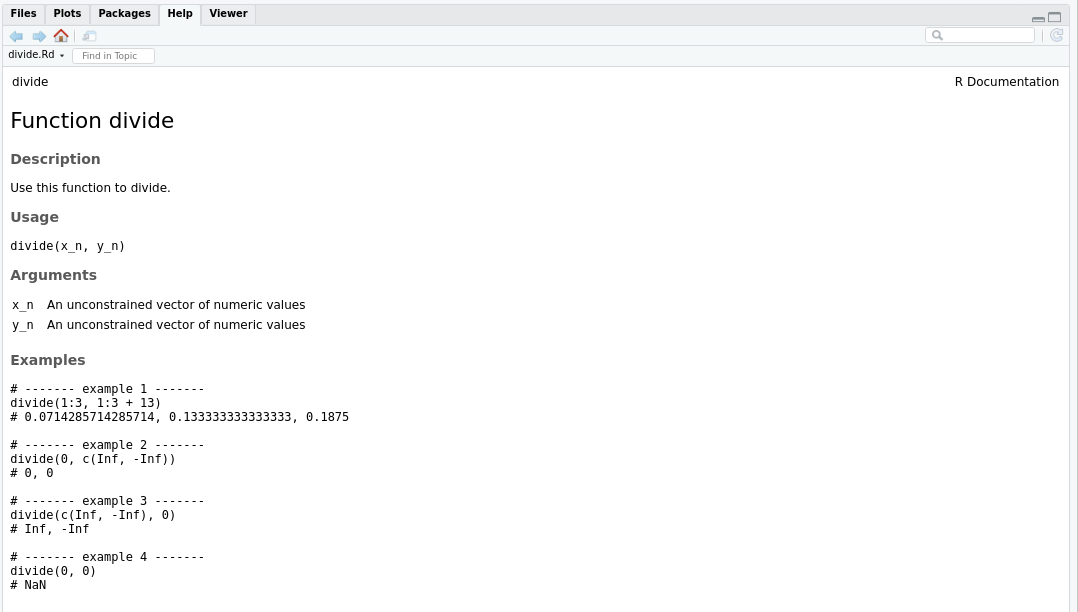This use-case vignette is dedicated to some common manual page use case generation using package wyz.code.rdoc.

It may make sense for newcommers to read the tutorial vignette prior to read this vignette.

# 1 Tips and tricks about R code

## 1.1 Parameter naming

Unless you specify a length of 1 or 1l, pluralize the parameter name. For example, avoid countryFlag_b_3, prefer countryFlags_b_3. Reason is quite simple, with the first one, you will have to correct produce documentation text as it is less likely to be correct in singular form than pluralized. When using no length specification, pluralizing that parameter name is the best practice. Understanding following examples, worth the time.

wyz.code.rdoc:::getTypeLabel(FunctionParameterName('countryFlag_b_3')) # wrong
 "a length-3 \\emph{\\code{vector}} of \\emph{\\code{boolean}} values representing the country flag"

wyz.code.rdoc:::getTypeLabel(FunctionParameterName('countryFlags_b_3')) # right
 "a length-3 \\emph{\\code{vector}} of \\emph{\\code{boolean}} values representing the country flags"
wyz.code.rdoc:::getTypeLabel(FunctionParameterName('countryFlags_b_1')) # wrong
 "a single \\emph{\\code{boolean}} value representing the country flags"

wyz.code.rdoc:::getTypeLabel(FunctionParameterName('countryFlag_b_1')) # right
 "a single \\emph{\\code{boolean}} value representing the country flag"
wyz.code.rdoc:::getTypeLabel(FunctionParameterName('countryFlag_b')) # wrong
 "an unconstrained \\emph{\\code{vector}} of \\emph{\\code{boolean}} values representing the country flag"

wyz.code.rdoc:::getTypeLabel(FunctionParameterName('countryFlags_b')) # right
 "an unconstrained \\emph{\\code{vector}} of \\emph{\\code{boolean}} values representing the country flags"

# 2 Tips and tricks about sections

Some sections have to be unique title, description, examples, … some others not keyword, concept, alias, …. You must respect the implicit contract of the standard R documentation. See writing R extensions for more information.

To add a section, just set its content to the one you desired. Content may contain format directives or not. See below, paragraph ‘about-format’.

My only advice is to keep content as simple and sharp as possible. Using non ambiguous terms and clear sentences helps a lot.

For example, to add a details section and three concept sections, you could do something similar to

pc <- ProcessingContext(
extraneous_l = list(
details = 'It is worth to know bla bla bla',
concept = paste0('concept-', 1:3)
)
)

## 2.2 complete a section

Activate post processing for sections you want to complete. For example, to complete the title section, you could do

pc <- ProcessingContext(
postProcessing_l = list(
title =  function(content_s) {
paste(content_s, sentensize('some complimentary content'))
}
)
)

## 2.3 remove a section

Just set its content to NULL.

pc <- ProcessingContext(
postProcessing_l = list(
details = function(content_s) NULL
)
)

# 3 Tips and tricks about content

## 3.1 content escaping

Content escaping is sometimes fully necessary, sometimes partially necessary and sometimes unneeded. Quite difficult to have a systematic approach, as content varies at lot according to section intent, section nature code, text, equation, … and also according to surrounding context.

To ease handling of content escape, wyz.code.rdoc offers several functions: a high-level function generateMarkup and a low-level function escapeContent.

By default content used is only partially escaped. Characters, ‘@’ and ‘%’ are systematically escaped, but not characters ‘{’ and ‘}’. To escape those last, you must set argument escapeBraces_b_1 to TRUE while using one or the other of those functions.

content <- 'function(x) { x + 1 }'

# To be use in a code section content
generateMarkup(content)
 "function(x) { x + 1 }"

# To be used in a text section content
paste('Some R code:', generateMarkup(content, escapeBraces_b_1 = TRUE))
 "Some R code: function(x) \\{ x + 1 \\}"

## 3.2 Content generation for R documentation section

As a end-user you should rely on use cases. As a programmer, you may need to create your own generation scheme to fulfil some special requirements. Following function could be useful to do so.

function name intent
generateSection generate a R documentation section
generateParagraph generate a paragraph collating all your inputs with a single new line by default.
generateParagraphCR generate a paragraph collating all your inputs with ‘\cr’
generateParagraph2NL generate a paragraph collating all your inputs with two new lines.

## 3.3 Content generation for examples section

Examples are a really important part of the documentation. It is also a quite tricky part when handcrafting documentation. This is due an inherent complexity related to contextual processing that has to take into consideration, testing time, necessary testing resources, test execution path, and so on.

In order to increase productivity and simplify the examples section, wyz.code.rdoc provides a dedicated function that turns pure R code into content.

Here is the pattern to follow.

1. create a variable that holds a list of functions taking no arguments. The body of each function must be legal R code, embodying the example
2. use function convertExamples to convert examples. You have the opportunity to pass along some keywords in order to manage test that should not be ran, should not be tested, should not be shown. You also have the opportunity to capture the example output and to introduce it automatically into the content.

Let’s see a sample session to do so

### 3.3.1 code

# The function to test
divide <- function(x_n, y_n) x_n / y_n

# The examples to consider
examples <- list(
function() { divide(1:3, 1:3 + 13L) },
function() { divide(0L, c(Inf, -Inf)) },
function() { divide(c(Inf, -Inf), 0L) },
function() { divide(0L, 0L) }
)

# your documentation complementary parts to consider
# and manual page generation context setup
ic <- InputContext(NULL, 'divide')
pc <- ProcessingContext(
extraneous_l = list(
examples = convertExamples(examples, captureOutput_b_1n = TRUE)
)
)
gc <- GenerationContext(tempdir(), overwrite = TRUE)

# The generation of the manual page
rv <- produceManualPage(ic, pc, gc)
File /tmp/RtmpxdfyT6/divide.Rd passes standard documentation checks 

### 3.3.2 content generated

readLines(rv$context$filename)
 "\\name{divide}"
 "\\alias{divide}"
 "\\title{Function divide}"
 "\\description{"
 "Use this function to divide."
 "}"
 "\\usage{"
 "divide(x_n, y_n)"
 "}"
 "\\arguments{"
 "\\item{x_n}{an unconstrained \\emph{\\code{vector}} of \\emph{\\code{numeric}} values representing the x}"
 "\\item{y_n}{an unconstrained \\emph{\\code{vector}} of \\emph{\\code{numeric}} values representing the y}"
 "}"
 "\\examples{"
 "# ------- example 1 -------"
 "divide(1:3, 1:3 + 13)"
 "# 0.0714285714285714, 0.133333333333333, 0.1875 "
 ""
 "# ------- example 2 -------"
 "divide(0, c(Inf, -Inf))"
 "# 0, 0 "
 ""
 "# ------- example 3 -------"
 "divide(c(Inf, -Inf), 0)"
 "# Inf, -Inf "
 ""
 "# ------- example 4 -------"
 "divide(0, 0)"
 "# NaN "
 ""
 "}"
 "\\keyword{function}"
 "\\encoding{UTF-8}"                                                                                        

### 3.3.3 generated content as picturegenerated content

# 4 Tips and tricks about format

## 4.1 enumeration format

Function generateEnumeration eases enumeration management.

generateEnumeration(paste('case', 1:4))
 "\\enumerate{\\item  case 1\n\\item  case 2\n\\item  case 3\n\\item  case 4}"

## 4.2 list format

Function generateEnumeration also eases item list management.

generateEnumeration(paste('case', 1:4), TRUE)
 "\\itemize{\\item  case 1\n\\item  case 2\n\\item  case 3\n\\item  case 4}"

## 4.3 table format

To format a table, use function generateTable.

dt <- data.table::data.table(x = paste0('XY_', 1:3), y = letters[1:3])

# as-is
generateTable(dt)
 "\\tabular{ll}{x \\tab y\\cr\n\nXY_1 \\tab a \\cr\nXY_2 \\tab b \\cr\nXY_3 \\tab c \\cr\n}"

# with row numbering
generateTable(dt, numberRows_b_1 = TRUE)
 "\\tabular{rll}{x \\tab y\\cr\n\n1 \\tab XY_1 \\tab a \\cr\n2 \\tab XY_2 \\tab b \\cr\n3 \\tab XY_3 \\tab c \\cr\n}"

## 4.4 special formats

Specification of R documentation is quite complex. There are many variants possible and many ways to achieve a result. Following functions try to provide one convenient solution for some common needs.

function name intent
generateOptionLink When you need to generate a cross package documentation link use this function. If you need an intra package documentation link use function beautify()$link. You could also use producePackageLink to generate a cross package documentation link, but you won’t be able to customize the labels. generateOptionSexpr When you need to generate a Sexpr, use generateMarkup when you don’t need options, otherwise use funciton generateOptionSexpr. generateEnc generate a locale text encoding and ASCII equivalence. Not to be confused with generateEncoding that set encoding for the full manual page. generateReference generate the text for a documentary or web reference. Refer to dedicated manual pages for more information. # 5 Tips and tricks about presentation Many typographic enhancements are available. They are all grouped behind a facade name beautify. b <- beautify() names(b)  "acronym" "bold" "cite" "code"  "dQuote" "email" "emph" "enc"  "env" "figure" "file" "format"  "kbd" "link" "option" "pkg"  "preformatted" "sQuote" "samp" "source"  "strong" "url" "var" "verb"  "codeLink" "enhanceCodeLink" "italicCode" "boldCode"  "R" "ldots" "dots"  b$bold('lorem ipsum')
 "\\bold{lorem ipsum}"
b$file('/tmp/result.txt')  "\\file{/tmp/result.txt}" b$acronym('CRAN')
 "\\acronym{CRAN}"
co <- '{ x %% y }'
b$code(co) # very probably wrong  "\\code{{ x \\%\\% y }}" e <- beautify(TRUE) e$code(co) # much more probably right
 "\\code{\\{ x \\%\\% y \\}}"

and the very convenient

# link to another package
b$code(producePackageLink('ggplot2', 'aes_string'))  "\\code{\\link[ggplot2:aes_string]{ggplot2:aes_string}}" # link to same package b$codeLink('generateTable')
 "\\emph{\\bold{\\code{\\link{generateTable}}}}"